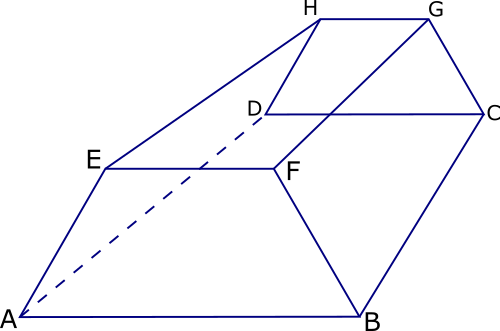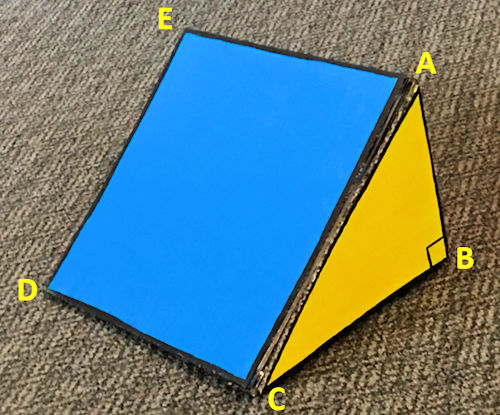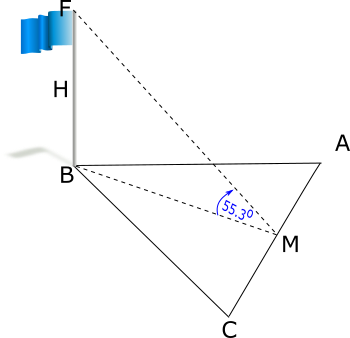# Exam-Style Questions on Trigonometry 3D

## Problems on Trigonometry 3D adapted from questions set in previous Mathematics exams.

### 1.

GCSE Higher

Rectangle ABCD is the horizontal base of a trapezoidal prism ABCDEFGH.

EF is parallel to AB. BC = 20cm, DC = 150mm, GC = 74mm, HG = 86mm and AE = BF.(a) Find the size of angle ABF.

(b) Show that FD = 242 mm correct to 3 significant figures.

### 2.

GCSE Higher

A triangular prism is made using two right-angled triangles and three rectangular pieces of cardboard. Five of the vertices have been labeled $$A, B, C, D \text{ and } E$$ as shown in the photograph below.$$AB = 17cm, \quad BC = 24cm, \quad DC = 28cm$$

Imagine a point $$F$$ on the line $$DC$$ such that:

$$DF : FC = 3 : 4$$

Calculate the size of the angle between $$AF$$ and the base of the prism.

### 3.

IB Studies

ABC is a triangular car park on horizontal ground. The length of AB is 90m and the length of AC is 65m. The size of angle BCA is 68o.

(a) Find the size of angle ABC.

(b) Find the area of the triangular car park.

M is the midpoint of AC.

(c) Find the length of BM.

A vertical flag pole, FB, is erected in one corner of the car park with its base at B.The angle of elevation of F from M is 55.3o. H is the midpoint of the flag pole.

(d) Calculate the angle of elevation of H from M.

If you would like space on the right of the question to write out the solution try this Thinning Feature. It will collapse the text into the left half of your screen but large diagrams will remain unchanged.

The exam-style questions appearing on this site are based on those set in previous examinations (or sample assessment papers for future examinations) by the major examination boards. The wording, diagrams and figures used in these questions have been changed from the originals so that students can have fresh, relevant problem solving practice even if they have previously worked through the related exam paper.

The solutions to the questions on this website are only available to those who have a Transum Subscription.

Exam-Style Questions Main Page

Search for exam-style questions containing a particular word or phrase:

To search the entire Transum website use the search box in the grey area below.